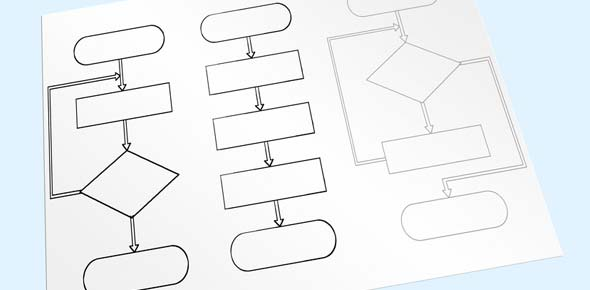# Assessment 1

13 Questions | Total Attempts: 45SettingsThis Assessment is for a Review of Addition Standard algorithms

Related Topics
• 1.
What do you do if numbers in a column add up to 10 or more?
• A.

Write the number under the line below without doing anything

• B.

Carry the tens place into the next column and write the ones place under the line below.

• C.

Ignore the number: it doesn't mean anything

• D.

Add the tens place and the ones place together, and write it under the line below

• E.

Write the ones place into the next column, and write the tens place on the lines below

• 2.
What does 12345+67890=? Please use a scratch piece of paper to complete the standard algorithm. YOU MAY NOT USE A CALCULATOR!
• A.

98653

• B.

98652

• C.

80234

• D.

80235

• E.

NONE OF THE ABOVE

• 3.
What is the answer to problem 1?
• A.

975

• B.

1000

• C.

980

• D.

890

• E.

976

• 4.
What is the Answer to problem 2?
• A.

208

• B.

207

• C.

209

• D.

211

• E.

210

• 5.
What is the Answer to Problem 3?
• A.

1619

• B.

1700

• C.

1789

• D.

1620

• E.

1790

• 6.
What is the answer to Problem 4?
• A.

910

• B.

909

• C.

911

• D.

908

• E.

960

• 7.
What is the answer to problem 5?
• A.

450

• B.

500

• C.

345

• D.

333

• E.

433

• 8.
What is the answer to problem 6?
• A.

500

• B.

403

• C.

303

• D.

503

• E.

603

• 9.
What is the answer to problem 7?
• A.

1275

• B.

1375

• C.

1385

• D.

1280

• E.

1276

• 10.
What is the anser to problem 8?
• A.

400

• B.

401

• C.

501

• D.

500

• E.

601

• 11.
What is the answer to problem 9?
• A.

813

• B.

820

• C.

713

• D.

1013

• E.

913

• 12.
What is the answer to problem 10?
• A.

500

• B.

657

• C.

557

• D.

657

• E.

457

• 13.
What do you do if when you add up the column farthest to the left, and it adds up 10 or more?
• A.

Write the number out normally below the line

• B.

Ignore this number: it doesn't mean anything!

• C.

Write only the ones place in the space below

• D.

Write only the tens place in the space below

• E.

Add the ones and tens space together and place it in the space below